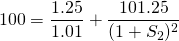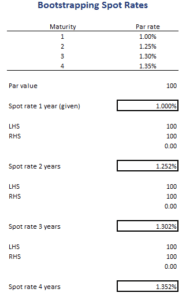# Bootstrapping Spot Rates

Bootstrapping spot rates using the par curve is a very important method that allows investors to derive zero coupon interest rates from the par rate curve. Bootstrapping the zero coupon yield curve is a step-by-step process that yields the spot rates in a sequential way. That is, we first obtain the spot rate for one year. Once we have that spot rate for one year, we can use it to obtain the spot rate for two years, and so on.

On this page, we discuss bootstrapping interest rate curves using the par rate curve. We also provide a bootstrapping example as well as a bootstrapping yield curve Excel spreadsheet that allows you to implement the approach with any set of par rates. The spreadsheet can be downloaded below.

## Bootstrapping Discount factors

Bootstrapping spot rates or zero coupon interest rates works as follows. Suppose we are given two par rates, the par rate for one year (1.00%) and the par rate for two years (1.25%). First, note that we generally know the spot rate for a one year zero-coupon bond because that is simple the one-year par rate. To get the spot rate for a two-year zero coupon bond, we use the following formulaNote that we set the left-hand side equal to 100. That’s because we are using the par curve. Solving the above formula, we obtain an interest rate of 1.252%. We can continue this process to calculate the 3-year zero coupon rate. This is something we illustrate in the numerical example below.

## Bootstrapping yield curve example

Let’s turn to an example bootstrapping the yield curve in Excel. In particular, let’s bootstrap the rates for up to four years in the future. The following table shows the necessary steps. The spreadsheet can be downloaded below. Note that we used the Solver function of Excel to solve for the spot rate. Alternatively, it is possible to algebraically solve for the unknown spot rate. While both approaches will yield identical results, it is generally faster to use the solver.It is important to understand that, to calculate the spot rate for a certain maturity, we first need to calculate the spot rates in all of the previous years first. That’s why the approach is called ‘bootstrapping’.

## Summary

We discussed bootstrapping the zero coupon yield curve using the par curve. The approach is very simple and allows bootstrapping discount factors. These discount factors can then be used to determine the present value of cash flows.

### Bootstrapping using par curve

Want to have an implementation in Excel? Download the Excel file: Bootstrapping Rates